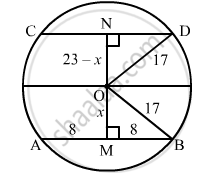Advertisement Remove all ads

# In a Circle of Radius 17 Cm, Two Parallel Chords Are Drawn on Opposite Side of Diameter. the Distance Between the Chords is 23 Cm. If the Length of One Chord is 16 Cm, Then the Length of the Other is - Mathematics

MCQ

In a circle of radius 17 cm, two parallel chords are drawn on opposite side of a diameter. The distance between the chords is 23 cm. If the length of one chord is 16 cm, then the length of the other is

#### Options

• 34 cm

• 15 cm

• 23 cm

• 30 cm

Advertisement Remove all ads

#### Solution

30 cm

Given that: Radius of the circle is 17 cm, distance between two parallel chords AB and CD is 23 cm, where AB= 16 cm. We have to find the length of CD.We know that the perpendicular drawn from the centre of the circle to any chord divides it into two equal parts.

So, AM = MB = 8 cm

Let OM = x cm

In triangle OMB,

x = sqrt(17^2 - 8^2 = 15)

Now, in triangle OND, ON = (23 − x) cm = (23 − 15) cm = 8 cm

ND = sqrt(OD^2 -ON^2)

⇒ ND = sqrt(17^2 - 8^2 = 15)

Therefore, the length of the other chord is

CD = 2 xx 15 = 30   cm

Is there an error in this question or solution?
Advertisement Remove all ads

#### APPEARS IN

RD Sharma Mathematics for Class 9
Chapter 15 Circles
Q 29 | Page 112
Advertisement Remove all ads

#### Video TutorialsVIEW ALL 

Advertisement Remove all ads
Share
Notifications

View all notifications

Forgot password?
Course